# 英雄哪里出来

## 夜深人静写算法（五） - 初等数论

1、整除性
2、素数
a.素数与合数
b.素数判定
c.素数定理
d.素数筛选法
3、因数分解
a.算术基本定理
b.素数拆分
c.因子个数
d.因子和
4、最大公约数(GCD)和最小公倍数(LCM)
5、同余
a.模运算
b.快速幂取模
c.循环节

1、欧几里德算法(辗转相除法)
2、扩展欧几里德定理
a.线性同余
b.同余方程求解
c.逆元
3、中国剩余定理（孙子定理）
4、欧拉函数
a.互素
b.筛选法求解欧拉函数
c.欧拉定理和费马小定理
5、容斥原理

1、Rabin-Miller 大素数判定
2、Pollard-rho 大数因式分解
3、RSA原理

1、整除性
若a和b都为整数，a整除b是指b是a的倍数，a是b的约数（因数、因子），记为a|b。整除的大部分性质都是显而易见的，为了阐述方便，我给这些性质都随便起了个名字。
i)  任意性，若a|b，则对于任意非零整数m，有am|bm。
ii) 传递性，若a|b，且b|c，则a|c。
iii) 可消性，若a|bc，且a和c互素(互素的概念下文会讲到)，则a|b。
iv) 组合性，若c|a，且c|b，则对于任意整数m、n，有c|(ma+nb)。
拿一个我还未出生时的初二数学竞赛题就能概括整除的性质了。
【例题1】(公元1987年初二数学竞赛题) x，y，z均为整数，若11｜（7x+2y-5z），求证：11｜（3x-7y+12z）。
非常典型的一个问题，为了描述方便，令a = （7x+2y-5z），b = （3x-7y+12z），通过构造可以得到一个等式：4a + 3b = 11（3x-2y+3z），则3b = 11（3x-2y+3z） - 4a。
任意性+组合性，得出 11 |（11（3x-2y+3z） - 4a） = 11|3b。
可消性，由于11和3互素，得出 11 | b，证明完毕。
2、素数
a.素数与合数
素数又称质数，素数首先满足条件是要大于等于2，并且除了1和它本身外，不能被其它任何自然数整除；其它的数称为合数；而1既非素数也非合数。
b.素数判定
如何判定一个数是否为素数？
i)  对n做[2, n)范围内的余数判定（C++中的'%'运算符），如果有至少一个数用n取余后为0，则表明n为合数；如果所有数都不能整除n，则n为素数，算法复杂度O(n)。
ii) 假设一个数能整除n，即a|n，那么n/a也必定能整除n，不妨设a <= n/a，则有a^2 <= n，即a <= sqrt(n)（sqrt表示对n开根号），所以在用i)的方法进行取余的时候，范围可以缩小到sqrt(n)，所以算法复杂度降为O( sqrt(n) )。
iii) 如果n是合数，那么它必然有一个小于等于sqrt(n)的素因子，只需要对sqrt(n)内的素数进行测试即可，需要预处理求出sqrt(n)中的素数，假设该范围内素数的个数为s，那么复杂度降为O(s)。
c.素数定理
当x很大时，小于x的素数的个数近似等于x/ln(x)，其中ln(x)表示x的自然对数，用极限表示如图一-2-1所示：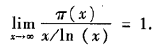从这个定理可以发现，程序中进行素数判定的时候，用ii)方法和iii)方法差了至少一个数量级。
d.素数筛选法
【例题2】给定n(n < 10000)个数，范围为[1, 2^32)，判定它是素数还是合数。
首先1不是素数，如果n>1，则枚举[1，sqrt(n)]范围内的素数进行试除，如果至少有一个素数能够整除n，则表明n是合数，否则n是素数。
[1，sqrt(n)]范围内的素数可以通过筛选法预先筛出来，用一个数组notprime[i]标记i是素数与否，筛选法有很多，这里介绍一种最常用的筛选法——Eratosthenes筛选法。
直接给出伪代码：
#define MAXP 65536
#define LL __int64
void Eratosthenes() {
notprime[1= true;
primes[0= 0;
for(int i = 2; i < MAXP; i++) {
if!notprime[i] ) {
primes[ ++primes ] = i;
//需要注意i*i超出整型后变成负数的问题，所以转化成 __int64
for(LL j = (LL)i*i; j < MAXP; j += i) {
notprime[j] = true;
}
}
}
}
notprime[i]为真表明i为合数，否则i为素数（因为全局变量初始值为false，筛选法预处理只做一次，所以不需要初始化）。算法的核心就是不断将notprime[i]标记为true的过程，首先从小到大进行枚举，遇到notprime[i]为假的，表明i是素数，将i保存到数组primes中，然后将i的倍数都标记为合数，由于i*2、i*3、i*(i-1)在[1, i)的筛选过程中必定已经被标记为合数了，所以i的倍数只需要从i*i开始即可，避免不必要的时间开销。
虽然这个算法有两个嵌套的轮询，但是第二个轮询只有在i是素数的时候才会执行，而且随着i的增大，它的倍数会越来越少，所以整个算法的时间复杂度并不是O(n^2)，而且远远小于O(n^2)，在notprime进行赋值的时候加入一个计数器count，计数器的值就是该程序的总执行次数，对MAXP进行不同的值测试发现 int(count / MAXP) 的值随着MAXP的增长变化非常小，总是维持在2左右，所以这个算法的复杂度可以近似看成是O(n)，更加确切的可以说是O(nC)，其中C为常数，C一般取2。
事实上，实际应用中由于空间的限制（空间复杂度为O(n)），MAXP的值并不会取的很大，10^7基本已经算是极限了，再大的素数测试就需要用到Rabin-Miller
（第三章中会介绍该算法的具体实现）大数判素了。
3、因数分解
a、算术基本定理
算术基本定理可以描述为：对于每个整数n，都可以唯一分解成素数的乘积，如图一-3-1所示：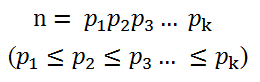这里的素数并不要求是不一样的，所以可以将相同的素数进行合并，采用素数幂的乘积进行表示，如图一-3-2所示：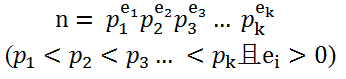证明方法采用数学归纳法，此处略去。
b、素数拆分
给定一个数n，如何将它拆分成素数的乘积呢？
还是用到上面讲到的试除法，假设 n = pm 并且 m>1，其中p为素数，如果p > sqrt(n)，那么根据算数基本定理，m中必定存在一个小于等于sqrt(n)的素数，所以我们不妨设p <= sqrt(n)。
然后通过枚举[2, sqrt(n)]的素数，如果能够找到一个素数p，使得n mod p == 0（mod 表示取余数、也称为模）。于是m = n/p，这时还需要注意一点，因为m中可能也有p这个素因子，所以如果p|m，需要继续试除，令m' = m/p，直到将所有的素因子p除尽，统计除的次数e，于是我们得到了 n = (p^e) * n'，然后继续枚举素数对n'做同样的试除。
枚举完[2, sqrt(n)]的素数后，得到表达式如图一-3-3所示：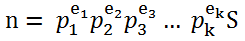这时有两种情况：
i)  S == 1，则素数分解完毕；
ii) S > 1, 根据算术基本定理，S 必定为素数，而且是大于sqrt(n)的素数，并且最多只有1个，这种情况同样适用于n本身就是素数的情况，这时n = S。
这样的分解方式称为因数分解，各个素因子可以用一个二元的结构体来存储。算法时间复杂度为O( s )，s为sqrt(n)内素数的个数。
c、因子个数
朴素的求因子个数的方法为枚举[1, n]的数进行余数判定，复杂度为O(n)，这里加入一个小优化，如果m为n的因子，那么必然n/m也为n的因子，不妨设m <= n/m，则有m <= sqrt(n)，所以只要枚举从[1, sqrt(n)]的因子然后计数即可，复杂度变为O(sqrt(n))。
【例题3】给定X,Y(X, Y < 2^31)，求X^Y的因子数 mod 10007。
由于这里的X^Y已经是天文数字，利用上述的枚举法已经无法满足要求，所以我们需要换个思路。考虑到任何整数都能表示成素数的乘积，那么X^Y也不例外，我们首先将X进行因数分解，那么X^Y可以表示成图一-3-4所示的形式：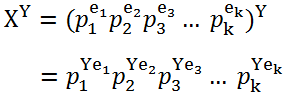容易发现X^Y的因子一定是p1、p2、...、pk的组合，并且p1可以取的个数为[0, Ye1]，p2可以取的个数为[0, Ye2]，pk可以取的个数为[0, Yek]，所以根据乘法原理，总的因子个数就是这些指数+1的连乘，即(1 + Ye1) * (1 + Ye2) * ... * (1 + Yek)。
通过这个问题，可以得到更加一般的求因子个数的公式，如果用ei表示X分解素因子之后的指数，那么X的因子个数就是(1 + e1) * (1 + e2) * ... * (1 + ek)。
d、因子和
【例题4】给定X,Y(X, Y < 2^31)，求X^Y的所有因子之和 mod 10007。
同样还是将X^Y表示成图一-3-4的形式，然后就变成了标准素数分解后的数的因子和问题了。考虑数n，令n的因子和为s(n)，对n进行素数分解后的，假设最小素数为p，素因子p的个数为e，那么n = (p^e)n'。
容易得知当n的因子中p的个数为0时，因子之和为s(n')。更加一般地，当n的因子中p的个数为k的时候，因子之和为(p^k)*s(n')，所以n的所有因子之和就可以表示成：
s(n) = (1 + p^1 + p^2 + ... p^e) * s(n') = (p^(e+1) - 1) / (p-1) * s(n')
s(n')可以通过相同方法递归计算。最后可以表示成一系列等比数列和的乘积。
令g(p, e) = (p^(e+1) - 1) / (p-1)，则s(n) = g(p1, e1) * g(p2, e2) * ... * g(pk, ek)。
4、最大公约数(GCD)和最小公倍数(LCM)
两个数a和b的最大公约数(Greatest Common Divisor)是指同时整除a和b的最大因数，记为gcd(a, b)。特殊的，当gcd(a, b) = 1，我们称a和b互素（上文谈到整除的时候略有提及）。
两个数a和b的最小公倍数(Leatest Common Multiple)是指同时被a和b整除的最小倍数，记为lcm(a, b)。特殊的，当a和b互素时，lcm(a, b) = ab。
gcd是基础数论中非常重要的概念，求解gcd一般采用辗转相除法（这个方法会在第二章开头着重介绍，这里先引出概念），而求lcm需要先求gcd，然后通过lcm(a, b) = ab / gcd(a, b)求解。
这里无意中引出了一个恒等式：lcm(a, b) * gcd(a, b) = ab。这个等式可以通过算术基本定理进行证明，证明过程可以通过图一-4-1秒懂。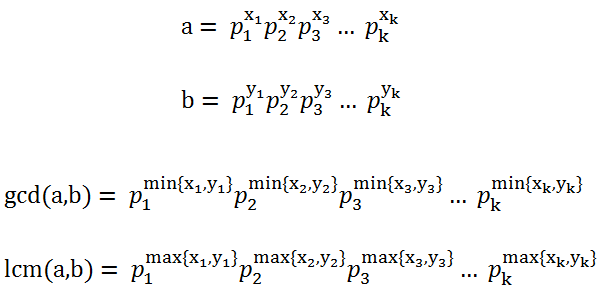需要说明的是这里的a和b的分解式中的指数是可以为0的，也就是说p1是a和b中某一个数的最小素因子，p2是次小的素因子。lcm(a, b)和gcd(a, b)相乘，相当于等式右边的每个素因子的指数相加，即min{xi, yi} + max{xi, yi} = xi + yi，正好对应了a和b的第i个素数分量的指数之和，得证。
给这样的gcd和lcm表示法冠个名以便后续使用——指数最值表示法。
【例题5】三个未知数x, y, z，它们的gcd为G，lcm为L，G和L已知，求(x, y, z)三元组的个数。
三个数的gcd可以参照两个数gcd的指数最值表示法，只不过每个素因子的指数上是三个数的最值（即min{x1, y1, z1}），那么这个问题首先要做的就是将G和L分别进行素因子分解，然后轮询L的每个素因子，对于每个素因子单独处理。
假设素因子为p，L分解式中p的指数为l，G分解式中p的指数为g，那么显然l < g时不可能存在满足条件的三元组，所以只需要讨论l >= g的情况，对于单个p因子，问题转化成了求三个数x1, y1, z1，满足min{x1, y1, z1} = g且max{x1, y1, z1} = l，更加通俗的意思就是三个数中最小的数是g，最大的数是l，另一个数在[g, l]范围内，这是一个排列组合问题，三元组{x1, y1, z1}的种类数当l == g时只有1中，否则答案就是 6(l - g)。
最后根据乘法原理将每个素因子对应的种类数相乘就是最后的答案了。

5、同余
a、模运算
给定一个正整数p，任意一个整数n，一定存在等式n = kp + r； 其中k、r是整数，且满足0 <= r < p，称k为n除以p的商， r为n除以p的余数，表示成n % p = r (这里采用C++语法，%表示取模运算)。
对于正整数和整数a, b, 定义如下运算：
取模运算：a % p（a mod p），表示a除以p的余数。
模p加法：(a + b) % p = (a%p + b%p) % p
模p减法：(a  - b) % p = (a%p  - b%p) % p
模p乘法：(a  * b) % p = ((a % p)*(b % p)) % p
幂模p   ： (a^b) % p = ((a % p)^b) % p
模运算满足结合律、交换律和分配律。
a≡b (mod n) 表示a和b模n同余，即a和b除以n的余数相等。
【例题6】一个n位十进制数(n <= 1000000)必定包含1、2、3、4四个数字，现在将它顺序重排，求给出一种方案，使得重排后的数是7的倍数。
取出1、2、3、4后，将剩下的数字随便排列得到一个数a，令剩下的四个数字排列出来的数为b，那么就是要找到一种方案使得(a*10000 + b) % 7等于0。
但是a真的可以随便排吗？也就是说如果无论a等于多少，都能找到这样的b满足等式成立，那么a就可以随便排。
我们将等式简化：
(a*10000 + b) % 7 = (a*10000%7 + b%7) % 7
令 k = a*10000%7 = a*4%7，容易发现k的取值为[0, 7)，如果b%7的取值也是[0, 7)，那这个问题就可以完美解决了，很幸运的是，的确可以构造出7个这样的b。具体参见下图：b、快速幂取模
幂取模常常用在RSA加密算法的加密和解密过程中，是指给定整数a，正整数n，以及非零整数p，求a^n % p。利用模p乘法，这个问题可以递归求解，即令f(n) = a^n%p，那么f(n-1) = a^(n-1)%p，f(n) = a*f(n-1) % p，这样就转化成了递归式。但是递归求解的时间复杂度为O(n)，往往当n很大的时候就很难在规定时间内出解了。
当n为偶数时，我们可以将a^n%p拆成两部分，令b = a^(n/2)%p，则a^n%p = b*b%p；
当n为奇数时，可以拆成三部分，令b = a^(n/2)%p，则a^n%p = a*b*b%p；
上述两个等式中的b可以通过递归计算，由于每次都是除2，所以时间复杂度是O(logn)。
c、循环节
【例题7】f = a, f = b, f = c, 当n>3时 f[n] = (A*f[n-1] + B*f[n-2] + C*f[n-3]) % 53，给定a, b, c, A, B, C，求f[n] (n < 2^31)。
由于n非常大，循环模拟求解肯定是不现实的，仔细观察可以发现当n>3时，f[n]的值域为[0, 53)，并且连续三个数f[n-1]、f[n-2]、f[n-3]一旦确定，那么f[n]也就确定了，而f[n-1]、f[n-2]、f[n-3]这三个数的组合数为53*53*53种情况，那么对于一个下标k<n，假设f[k]已经求出，并且满足f[k-1] == f[n-1]且f[k-2] == f[n-2]且f[k-3] == f[n-3],  则f[n]必定等于f[k]，这里的f[k...n-1]就被称为这个数列的循环节。
并且在53*53*53次计算之内必定能够找到循环节，这个是显而易见的。

1、欧几里德定理（辗转相除法）
定理：gcd(a, b) = gcd(b, a % b)。
证明：a = kb + r = kb + a%b，则a % b = a - kb。令d为a和b的公约数，则d|a且d|b 根据整除的组合性原则，有d|(a-kb)，即d|(a%b)。
这就说明如果d是a和b的公约数，那么d也一定是b和a%b的公约数，即两者的公约数是一样的，所以最大公约数也必定相等。
这个定理可以直接用递归实现，代码如下：
int gcd(int a, int b) {
return b ? gcd(b, a%b) : a;
}
这个函数揭示了一个约定俗成的概念，即任何非零整数和零的最大公约数为它本身。
【例题8】f = 0, 当n>1时，f[n] = (f[n-1]+a) % b，给定a和b，问是否存在一个自然数k (0 <= k< b)，是f[n]永远都取不到的。
永远有多远？并不是本题的范畴。
但是可以发现的是这里的f[...]一定是有循环节的，如果在某个循环节内都无法找到那个自然数k，那么必定是永远都找不到了。
求出f[n]的通项公式，为f[n] = an % b，令an = kb + r，那么这里的r = f[n]，如果t = gcd(a, b)，r = an-kb = t ( (a/t)n - (b/t)k )，则有t|r，要满足所有的r使得t|r,只有当t = 1的时候，于是这个问题的解也就出来了，只要求a和b的gcd，如果gcd(a, b) > 1，则存在一个k使得f[n]永远都取不到，直观的理解是当gcd(a, b) > 1，那么f[n]不可能是素数。

2、扩展欧几里德定理
a、线性同余
线性同余方程（也可以叫模线性方程）是最基本的同余方程，即ax≡b (mod n)，其中a、b、n都为常量，x是未知数，这个方程可以进行一定的转化，得到：ax = kn + b,这里的k为任意整数，于是我们可以得到更加一般的形式即：ax + by + c = 0，这个方程就是二维空间中的直线方程，但是x和y的取值为整数，所以这个方程的解是一些排列成直线的点集。
b、同余方程求解
求解同余方程第一步是转化成一般式：ax + by = c，这个方程的求解步骤如下：
i) 首先求出a和b的最大公约数d = gcd(a, b)，那么原方程可以转化成d(ax/d + by/d) = c，容易知道(ax/d + by/d)为整数，如若d不能整除b，方程必然无解，算法结束；否则进入ii)。
ii) 由i)可以得知，方程有解则一定可以表示成 ax + by = c = gcd(a, b)*c'，那么我们先来看如何求解d = gcd(a, b) = ax + by，根据欧几里德定理，有：
d = gcd(a, b) = gcd(b, a%b) = bx' + (a%b)y' = bx' + [a-b*(a/b)]y' = ay' + b[x' - (a/b)y']
于是有x = y',  y = x' - (a/b)y'。
由于gcd(a, b)是一个递归的计算，所以在求解(x, y)时，(x', y')其实已经利用递归计算出来了，递归出口为b == 0的时候（对比辗转相除，也是b == 0的时候递归结束），那么这时方程的解x0 = 1, y0 = 0。代码如下：
#define LL __int64
LL Extend_Euclid(LL a, LL b, LL &X, LL &Y) {
LL q, temp;
if!b ) {
X = 1; Y = 0;
return a;
}else {
q = Extend_Euclid(b, a % b, X, Y);
temp = X;
X = Y;
Y = temp - (a / b) * Y;
return q;
}
}
扩展欧几里德算法和欧几里德算法的返回值一致，都是gcd(a, b)，传参多了两个未知数X, Y，采用引用的形式进行传递，对应上文提到的x, y，递归出口为b == 0，这时返回值为当前的a，因为gcd(a, 0) = a，(X, Y)初值为(1, 0)，然后经过回溯不断计算新的(X, Y)，这个计算是利用了之前的(X, Y)进行迭代计算的，直到回溯到最上层算法终止。最后得到的(X, Y)就是方程gcd(a, b) = ax + by的解。
通过扩展欧几里德求的是ax + by = gcd(a, b)的解，令解为(x0, y0)，代入原方程，得：ax0 + by0 = gcd(a, b)，如果要求ax + by = c = gcd(a, b)*c'，可以将上式代入，得：ax + by = c = (ax0 + by0)c'，则x = x0c', y = y0c'，这里的(x, y)只是这个方程的其中一组解，x的通解为 { x0c' + kb/gcd(a, b) | k为任意整数 }，y的通解可以通过x通解的代入得出。
【例题9】有两只青蛙，青蛙A和青蛙B，它们在一个首尾相接的数轴上。设青蛙A的出发点坐标是x，青蛙B的出发点坐标是y。青蛙A一次能跳m米，青蛙B一次能跳n米，两只青蛙跳一次所花费的时间相同。数轴总长L米。要求它们至少跳了几次以后才会碰面。
假设跳了t次后相遇，则可以列出方程：(x + mt) % L = (y + nt) % L
将未知数t移到等式左边，常数移到等式右边，得到模线性方程：(m-n)t%L = (y-x)%L   （即 ax≡b (mod n) 的形式）
利用扩展欧几里德定理可以求得t的通解{ t0 + kd | k为任意整数 }，由于这里需要求t的最小正整数，而t0不一定是最小的正整数，甚至有可能是负数，我们发现t的通解是关于d同余的，所以最后的解可以做如下处理：ans = (t0 % d + d) % d。
c、逆元
模逆元的最通俗含义可以效仿乘法，a*x = 1，则称x为a在乘法域上的逆（倒数）；同样，如果ax≡1 (mod n)，则称b为a模n的逆，简称逆元。求a模n的逆元，就是模线性方程ax≡b (mod n)中b等于1的特殊形式，可以用扩展欧几里德求解。并且在gcd(a, n) > 1时逆不存在。
3、中国剩余定理
上文提到了模线性方程的求解，再来介绍一种模线性方程组的求解，模线性方程组如图二-3-1所示，其中(ai, mi)都是已知量，求最小的x满足以下n个等式：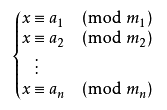将模数保存在mod数组中，余数保存在rem数组中，则上面的问题可以表示成以下几个式子，我们的目的是要求出一个最小的正整数K满足所有等式：
K = mod * x + rem     (0)
K = mod * x + rem     (1)
K = mod * x + rem     (2)
K = mod * x + rem     (3)
... ...
这里给出我的算法，大体的思想就是每次合并两个方程，经过n-1次合并后剩下一个方程，方程的自变量取0时得到最小正整数解。算法描述如下：
i)   迭代器i = 0
ii)  x[i] = (newMod[i]*k + newRem[i])            (k为任意整数)
iii) 合并(i)和(i+1)，得 mod[i] * x[i] - mod[i+1] * x[i+1] = rem[i+1] - rem[i]
将x[i]代入上式，有 newMod[i]*mod[i]*k - mod[i+1] * x[i+1] = rem[i+1] - rem[i] - newRem[i]*mod[i]
iv)  那么产生了一个形如 a*k + b*x[i+1] = c的同余方程，
其中a = newMod[i]*mod[i], b = - mod[i+1], c = rem[i+1] - rem[i] - newRem[i]*mod[i]
求解同余方程，如果a和b的gcd不能整除c，则整个同余方程组无解，算法结束；
否则，利用扩展欧几里德求解x[i+1]的通解，通解可以表示成 x[i+1] = (newMod[i+1]*k + newRem[i+1])            (k为任意整数)
v)  迭代器i++,如果i == n算法结束，最后答案为 newRem[n-1] * mod[n-1] + rem[n-1]；否则跳转到ii)继续迭代计算。
4、欧拉函数
a、互素
两个数a和b互素的定义为：gcd(a, b) = 1，那么如何求不大于n且与n互素的数的个数呢？
朴素算法，枚举i从1到n，当gcd(i, n)=1时计数器++，算法时间复杂度O(n)。
这里引入一个新的概念：用φ(n)表示不大于n且与n互素的数的个数，该函数以欧拉的名字命名，称为欧拉函数。
如果n是一个素数，即n = p，那么φ(n) = p-1（所有小于n的都互素）；
如果n是素数的k次幂，即n = p^k，那么φ(n) = p^k - p^(k-1) （除了p的倍数其它都互素）；
如果m和n互素，那么φ(mn) = φ(m)φ(n)（可以利用上面两个性质进行推导）。
将n分解成如图二-4-1的素因子形式，那么利用上面的定理可得φ(n)如图二-4-2所示：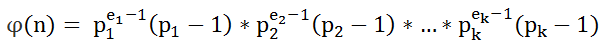前面已经讲到n的因子分解复杂度为O(k)，所以欧拉函数的求解就是O(k)。
b、筛选法求解欧拉函数
由于欧拉函数的表示法和整数的素数拆分表示法很类似，都可以表示成一些素数的函数的乘积，所以同样可以利用筛选法进行求解。伪代码如下：
#define MAXP 2000010
#define LL __int64
void Eratosthenes_Phi() {
notprime = true;
for(int i = 1; i < MAXP; i++) phi[i] = 1;
for(int i = 2; i < MAXP; i++) {
if( !notprime[i] ) {
phi[i] *= i - 1;
// 和传统素数筛法的区别在于这个i+i
for(int j = i+i; j < MAXP; j += i) {
notprime[j] = true;
int n = j / i;
phi[j] *= (i - 1);
while(n % i == 0) n /= i, phi[j] *= i;
}
}
}
}
这里的phi[i]保存了i这个数的欧拉函数，还是利用素数筛选将所有素数筛选出来，然后针对每个素因子计算它的倍数含有该素因子的个数，利用欧拉公式计算该素因子带来的欧拉函数分量，整个筛选过程可以参考素数筛选。
c、欧拉定理和费马小定理
欧拉定理：若n,a为正整数，且n,a互素，则: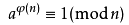费马小定理：若p为素数，a为正整数且和p互素，则：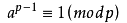由于当n为素数时φ(n) = p-1，可见费马小定理是欧拉定理的特殊形式。
证明随处可见，这里讲一下应用。
【例题10】整数a和n互素，求a的k次幂模n，其中k = X^Y, 正整数a,n,X,Y（X,Y<=10^9）为给定值。
问题要求的是a^(X^Y) % n，指数上还是存在指数，需要将指数化简，注意到a和n互素，所以可以利用欧拉定理，令X^Y = kφ(n) + r，那么kφ(n)部分并不需要考虑，问题转化成求r = X^Y % φ(n)，可以采用快速幂取模，二分求解，得到r后再采用快速幂取模求解a^r % n。
5、容斥原理
容斥原理是应用在集合上的，来看图二-5-1，要求图中两个圆的并面积，我们的做法是先将两个圆的面积相加，然后发现相交的部分多加了一次，予以减去；对于图二-5-2的三个圆的并面积，则是先将三个圆的面积相加，然后减去两两相交的部分，而三个圆相交的部分被多减了一次，予以加回。这里的“加”就是“容”，“减”就是“斥”，并且“容”和“斥”总是交替进行的（一个的加上，两个的减去，三个的加上，四个的减去），而且可以推广到n个元素的情况。
【例题11】求小于等于m(m < 2^31)并且与n(n < 2^31)互素的数的个数。
当m等于n，就是一个简单的欧拉函数求解。
但是一般情况m都是不等于n的，所以可以直接摈弃欧拉函数的思路了。
考虑将n分解成素数幂的乘积，来看一种最简单的情况，当n为素数的幂即n = p^k时，显然答案等于m - m/p（m/p表示的是p的倍数，去掉p的倍数，则都是和n互素的数了）；然后再来讨论n是两个素数的幂的乘积的情况，即n = p1^k1 * p2^k2，那么我们需要做的就是找到p1的倍数和p2的倍数，并且要减去p1和p2的公公倍数，这个思想其实已经是容斥了，所以这种情况下答案为：m - ( m/p1 + m/p2 - m/(p1*p2) )。
类比两个素因子，如果n分解成s个素因子，也同样可以用容斥原理求解。
容斥原理其实是枚举子集的过程，常见的枚举方法为dfs，也可以采用二进制法（0表示取，1表示不取）。这里给出一版dfs版本的容斥原理的伪代码，用于求解小于等于m且与n互素的数的个数。
#define LL __int64
void IncludeExclude(int depth, LL m, LL mul, int op, int* p, LL &ans) {
if(m < mul) return ;
if(depth == p) {
ans += (op ? -1 : 1) * (m / mul);
return ;
}
for(int i = 0; i < 2; i++) {
// 0 表示不取, 1表示取
IncludeExclude( depth+1, m, mul * (i?p[depth+1]:1), op^i, p, ans );
}
}
p[ 1 : p ]存储的是n的所有素因子，p表示数组长度，mul表示该次的素因子子集的乘积，op表示子集的奇偶性，ans存储最后的答案。
例如求[1, 9]中和6互素的数的个数，这时p = [2, 2, 3] （注意p是存素数的个数的，6分解的素因子为2和3）。

ans = 9/1 - （9/2 + 9/3） + 9/6 = 3，ans分为三部分，0个数的组合，1个数的组合，2个数的组合。

1、Rabin-Miller 大素数判定
对于一个很大的数n（例如十进制表示有100位），如果还是采用试除法进行判定，时间复杂度必定难以承受，目前比较稳定的大素数判定法是拉宾-米勒（Rabin-Miller）素数判定。
拉宾-米勒判定是基于费马小定理的，即如果一个数p为素数的条件是对于所有和p互素的正整数a满足以下等式：然而我们不可能试遍所有和p互素的正整数，这样的话和试除比算法的复杂度反而更高，事实上我们只需要取比p小的几个素数进行测试就行了。
具体判断n是否为素数的算法如下：
i)   如果n==2，返回true；如果 n<2|| !(n&1), 返回false；否则跳到ii)。
ii)  令n = m*（2^k） + 1，其中m为奇数，则n-1 = m*（2^k）。
iii) 枚举小于n的素数p（至多枚举10个），对每个素数执行费马测试，费马测试如下：计算pre = p^m % n，如果pre等于1，则该测试失效，继续回到iii)测试下一个素数；否则进行k次计算next = pre^2 % n，如果next == 1 && pre ！= 1 && pre != n-1则n必定是合数，直接返回；k次计算结束判断pre的值，如果不等于1，必定是合数。
iv) 10次判定完毕，如果n都没有检测出是合数，那么n为素数。
伪代码如下：
bool Rabin_Miller(LL n) {
LL k = 0, m = n-1;
if(n == 2) return true;
if(n < 2 || !(n & 1)) return false;
// 将n-1表示成m*2^k
while( !(m & 1) ) k++, m >>= 1;
for(int i = 0; i < 10; i++) {
if(p[i] == n)
return true;
if( isRealComposite(p[i], n, m, k) ) {
return false;
}
}
return true;
}
这里的函数isRealComposite(p, n, m, k)就是费马测试，p^(m*2^k) % n不等于1则n必定为合数，这是根据费马小定理得出的（注意）。n-1 = m*（2^k）
isRealComposite实现如下：
bool isRealComposite(LL p, LL n, LL m, LL k) {
LL pre = Power_Mod(p, m, n);
if(pre == 1) {
return false;
}
while(k--) {
LL next = Product_Mod(pre, pre, n);
if(next == 1 && pre != 1 && pre != n-1)
return true;
pre = next;
}
return ( pre != 1 );
}
这里Power_Mod(a, b, n)即a^b%n，Product_Mod(a, b, n)即a*b%n，而k次测试的基于费马小定理的一个推论：x^2 % n = 1当n为素数时x的解只有两个，即1和n-1。
2、Pollard-rho 大数因式分解

Pollard-rho的策略为：从[2, n)中随机选取k个数x1、x2、x3、...、xk，求任意两个数xi、xj的差和n的最大公约数，即d = gcd(xi - xj, n)，如果1 < d < n，则d为n的一个因子，直接返回d即可。
然后来看如何选取这k个数，我们采用生成函数法，令x1 = rand()%(n-1) + 1，xi = （xi-1 ^ 2 + 1 ) mod n，很明显，这个序列是有循环节的，就像图三-2-1那样。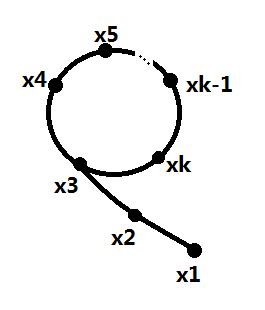我们需要做的就是在它进入循环的时候及时跳出循环，因为x1是随机选的，x1选的不好可能使得这个算法永远都找不到n的一个范围在(1, n)的因子，这里采用歩进法，保证在进入环的时候直接跳出循环，具体算法伪代码如下：
LL Pollard_rho(LL n) {
LL x = rand() % (n - 1) + 1;
LL y = x;
LL i = 1, k = 2;
do {
i++;
LL p = gcd(n + y - x, n);   // 这里传入的gcd需要是正数
if(1 < p && p < n) {
return p;
}
if(i == k) {
k <<= 1;
y = x;
}
x = Func(x, n);
}while(x != y);
return n;
}
3、RSA原理
RSA算法有三个参数，n、pub、pri，其中n等于两个大素数p和q的乘积(n = p*q)，pub可以任意取，但是要求与(p-1)*(q-1)互素，pub*pri % () = 1 (可以理解为pri是pub的逆元)，那么这里的(n, pub)称为公钥，(n, pri)称为私钥。(p-1)*(q-1)
RSA算法的加密和解密是一致的，令x为明文，y为密文，则：
加密：y = x ^ pub % n    (利用公钥加密，y = encode(x) )
解密：x = y ^ pri % n      (利用私钥解密，x = decode(y) )
那么我们来看看这个算法是如何运作的。
假设你得到了一个密文y，并且手上只有公钥，如何得到明文x，从decode的情况来看，只要知道私钥貌似就可以了，而私钥的获取方式只有一个，就是求公钥对(p-1)*(q-1)的逆元，如果(p-1)*(q-1)已知，那么可以利用扩展欧几里德定理进行求解，问题是(p-1)*(q-1)是未知的，但是我们有n = p*q，于是问题归根结底其实是难在了对n进行素因子分解上了，Pollard-rho的分解算法时间 复杂度只能达到O(n ^(1/4) )，对int64范围内的整数可以在几十毫秒内出解，而当n是几百位的大数的时候计算时间就只能用天来衡量了。

1、素数和因数分解
Largest prime factor   ★☆☆☆☆        素数筛选
The number of divisors ★☆☆☆☆        因子数
七夕节                  ★☆☆☆☆        因子和
Happy 2004             ★☆☆☆☆        X^Y的因子和
Number Sequence        ★☆☆☆☆        循环节的经典问题
Beijing 2008           ★★☆☆☆        X^Y的因子和
f(n)                   ☆☆☆        找规律+素数筛选
本原串                  ★★☆☆☆        整除性质 + 因子枚举
Special Prime          ★★☆☆☆        3n^2+3n+1 的素数判定问题
Factorial Simplificat  ★★★☆☆        因式分解+树状数组+DFS
Gcd & Lcm game         ★★☆        因式分解+线段树
2、GCD && LCM
hide handkerchief      ★☆☆☆☆        互素判定
GCD and LCM            ★★☆☆☆        GCD和LCM性质 + 排列组合
Revenge of GCD         ★★☆☆☆        辗转相除+因子枚举
Least common multiple  ★★☆☆        GCD性质 + 完全背包
3、同余性质 和 循环节
N!Again                ☆☆☆        同余的乘法性质
Ice Rain               ★★☆☆☆        余数性质
Love you TenThous years★★☆☆☆        规律
TCE-frep number system ★★☆☆☆        完全数
Perfect Squares        ★★☆☆☆        同余性 + 循环节
X mod f(x)             ★★☆☆        利用同余原理进行动态规划
Interesting Fibonacci  ★★        复杂的循环节
4、模线性方程和逆元
青蛙的约会               ★☆☆☆☆        线性同余
Romantic               ★☆☆☆☆        线性同余
Robot                  ★★☆☆☆        逆元
An easy problem        ★★☆☆☆        逆元
A/B                    ★★☆☆☆        逆元入门题
A New Change Problem   ★★☆☆☆        同余推导
Central Meridian Number★★☆☆☆        线性同余+枚举
number theory          ★★☆☆☆        快速幂取模 + 欧几里德定理
Multiply game          ★★★★☆        树状数组 + 逆元
5、模线性方程组
Chinese remainder theorem again ★★☆☆☆ 中国剩余定理 简化版
Strange Way to Express Integers ★★★☆☆ 中国剩余定理 模板题
Hello Kiki                      ★★★☆☆ 中国剩余定理 模板题
X问题                            ★★★☆☆ 中国剩余定理 模板题
And Now, a Remainder from       ★★★☆☆ 中国剩余定理 模板题

6、欧拉函数、欧拉定理、费马小定理
2^x mod n = 1           ★☆☆☆☆       费马小定理 简化版的
HeHe                    ★☆☆☆☆       欧拉函数
GCD                     ★★☆☆☆       欧拉函数
Become A Hero           ★★☆☆       筛选法求欧拉函数
The Euler function      ★★☆☆☆       筛选法求欧拉函数
The Luckiest number     ★★★☆☆       费马小定理
Calculation             ★★★☆☆       费马小定理
Description has only two Sentences  ★★★☆☆ 费马小定理，我的题
6、容斥原理
How many integers                ★★☆☆       容斥原理
Visible Trees           ★★☆☆☆       容斥原理
GCD Again               ★★☆☆☆       容斥原理
GCD                     ★★☆☆☆       容斥原理
GCD                     ★★☆☆☆       容斥原理
Coprime                 ★★★☆☆       二分枚举+容斥原理
Sky Code                ★★☆☆       容斥原理
7、大素数判定
GCD & LCM Inverse       ★★☆☆       拉宾米勒大数判素+dfs
Pseudoprime numbers     ★★☆☆       拉宾米勒
Prime Test              ★★★☆       拉宾米勒+Pollard-rho
RSA                     ★★★★☆       拉宾米勒 + 线性同余
8、离散对数-Baby Step Gaint Step算法
Discrete Logging        ★★☆☆       基础
Mod Tree                ★★☆       扩展Baby Step Giant Step
Matrix Puzzle           ★★       Baby Step Giant Step + 高斯消元
9、其它

posted on 2015-12-02 22:05 英雄哪里出来 阅读(14719) 评论(2)  编辑 收藏 引用 所属分类: 算法专辑## #re: 夜深人静写算法（五） - 初等数论[未登录]  回复更多评论

2015-12-04 14:56 | Wang

## #re: 夜深人静写算法（五） - 初等数论回复更多评论

C找循环节求递推式可以，也可以构造矩阵直接用矩阵快速幂啊~
2016-08-17 16:10 | kirai